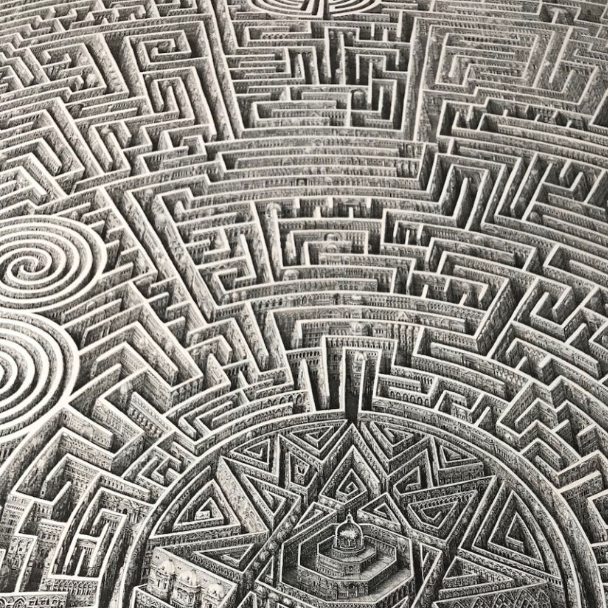Combinatorics

The additive dimension of a set, which is the size of a maximal dissociated subset, is closely connected to the rapid growth of higher sumsets.

## Additive dimension and the growth of sets

Submitted

I. Shkredov

We develop the theory of the additive dimension $\dim(A)$, i.e. the size of a maximal dissociated subset of a set $A$. It was shown that the additive dimension is closely connected with the growth of higher sumsets $nA$ of our set $A$. We apply this approach to demonstrate that for any small multiplicative subgroup $\Gamma$ the sequence $|n\Gamma|$ grows very fast. Also, we obtain a series of applications to the sum--product phenomenon and to the Balog--Wooley decomposition--type results.

Submitted

I. Shkredov